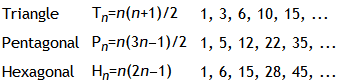# Project Euler – Problem 45 Solution

#### Problem

Triangle, pentagonal, and hexagonal numbers are generated by the following formulae:It can be verified that T285 = P165 = H143 = 40755.

Find the next triangle number that is also pentagonal and hexagonal.

#### Solution

```let naturalNumbers n = Seq.unfold (fun state -> Some(state, state + 1I)) n

// define the function T, P and H
let T n = n * (n + 1I) / 2I
let P n = n * (3I * n - 1I) / 2I
let H n = n * (2I * n - 1I)

// define the sequences for each function from the point the brief left off at
let TSeq = naturalNumbers 285I |> Seq.map T
let PSeq = naturalNumbers 165I |> Seq.map P
let HSeq = naturalNumbers 143I |> Seq.map H